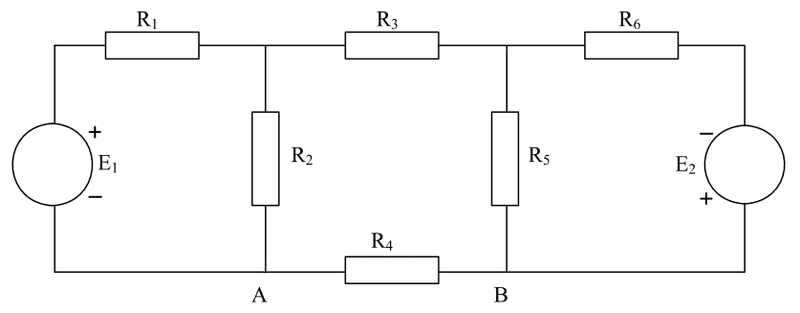# Thévenin resistance and Load resistance vs maximum power

## Homework StatementThis is the network.

The problem states that a new resistor is put between A and B, in parallel with R4.
I should find the ideal resistance for this load resistor and show that this is indeed the ideal resistance for maximum power output.

## Homework Equations

$$R_th = R_L-max$$
$$P=\frac{E_(th)^2}{4 \times R_th}$$

## The Attempt at a Solution

I have determined the Thévenin resistance and voltage in an earlier problem and found them to be:
Rth = 1.66 ohm
Eth = 8 Volts
I_R4 (before load resistor is connected) = 4 ampere
This is double checked with the solution.

I have also double checked that the load resistor should be 1.66 ohm to provide maximum power.

The power output is found using the P=formula stated above which gives me 9.36 W.
This is also correct according to the solution.

My problem is showing that with a lower or higher resistance, the power output will drop.
I can't simply put another resistance value into the formula above as the power will increase if I insert a lower resistance.

Can someone kick me a step in the right direction?

gneill
Mentor
Use the Thevenin equivalent circuit to drive a load resistor R of indeterminate value (it's a variable). Write the expression for the power transferred to R. How might you going about maximizing the power expression with respect to R?

When i draw the thevenin circiuit with load resistor connected i will have an 8V dc suppy in parallel with 1,66 ohm thevenin resistor in parallel with 1.66 ohm load resistor.

Using these numbers, i get that the effect in the load resistor is 38W (P=U^2 / R where u=8V and R = 1,66ohm)

So what am I not taking into concideration in this situation?

gneill
Mentor
A Thevenin equivalent consists of a voltage source in SERIES with a Thevenin resistance.

Oh my god.

Thank you.

That implies that even when the text said "a load resistor is connected to R4 in parallel", the load resistor is in series when doing the calculations based on the thevenin equivalents.

The text fooled me.